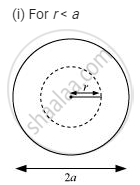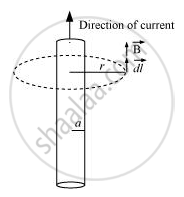PUC Karnataka Science Class 12Department of Pre-University Education, Karnataka
Share

# A Long Straight Wire of a Circular Cross-section of Radius ‘A’ Carries a Steady Current ‘I’. the Current is Uniformly Distributed Across the Cross-section. Apply Ampere’S Circuital Law to Calculate - PUC Karnataka Science Class 12 - Physics

#### Question

A long straight wire of a circular cross-section of radius ‘a’ carries a steady current ‘I’. The current is uniformly distributed across the cross-section. Apply Ampere’s circuital law to calculate the magnetic field at a point ‘r’ in the region for (i) r < a and (ii) r > a.

#### SolutionointvecB*vecdl = mu_0I_(enclosed)

(I_(enclosed))/(pia^2) = I/(pir^2)

I_(enclosed) = I r_2/a^2

vecB*vecdl = Bdl   (because costheta = 1)

therefore ointBdl = mu_0Ir_2/a^2

Bointdl = mu_0Ir^2/a^2

B(2pir) = mu_0 Ir^2/a^2

B = (mu_0)/(2pi) I/a^2  r

(ii) For r aFrom Ampere’s circuital law,

ointvecB*vecdl  = mu_0 l_(enclosed)

vecB*vecdl =Bdt cos theta

theta = 0

I_(enclosed) = I

oint Bdl = mu_0I

Bointdl = mu_0I

B(2pir) = mu_0I

B= (mu_0)/(2pi) I/r

Is there an error in this question or solution?

#### Video TutorialsVIEW ALL 

Solution A Long Straight Wire of a Circular Cross-section of Radius ‘A’ Carries a Steady Current ‘I’. the Current is Uniformly Distributed Across the Cross-section. Apply Ampere’S Circuital Law to Calculate Concept: Ampere’s Circuital Law.
S